Latest Banking jobs   »   Quantitative Aptitude Quiz For SBI PO,...

# Quantitative Aptitude Quiz For SBI PO,Clerk Pre 2021- 30th June

Direction (1-5): The given below pie chart shows the percentage distribution of daily consumption of quantity of water by five different families in a building. Read the pie-chart carefully and answer the following questions.
Total quantity of water consumed in a day =7,000 liters.
Note-Total quantity of water available = Total quantity of water consumed + total quantity of unused water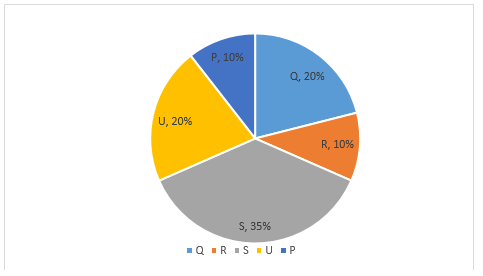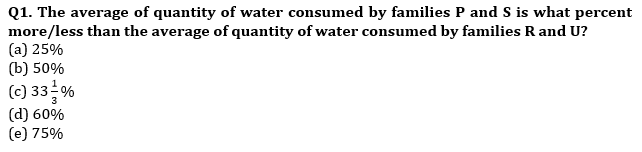Q2. If 87.5% of the quantity of water available is consumed by all families. Then, find the ratio of quantity of unused water to the difference of the quantity of water consumed by families S and Q?
(a) 6 : 7
(b) 44 : 45
(c) 62 : 63
(d) 20 : 21
(e) 14 : 15

Q3. Find the ratio of the quantity of water consumed by family S and U together to the quantity of water consumed by families P and R together?
(a) 11 : 4
(b) 5 : 4
(c) 3 : 2
(d) 13 : 8
(e) 15 : 8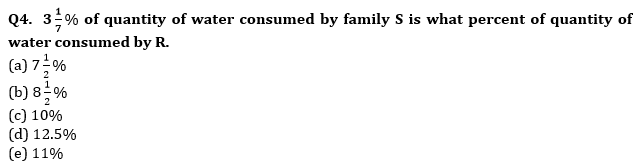Q5. The difference of the quantity of water consumed by families U and S is how much more than the difference of the quantity of water consumed by family Q and R?
(a) 350 liter
(b) 320 liter
(c) 330 liter
(d) 360 liter
(e) 340 liter

Directions (6-10): Given pie-chart shows the degree wise distribution of expenditure of India on different sports.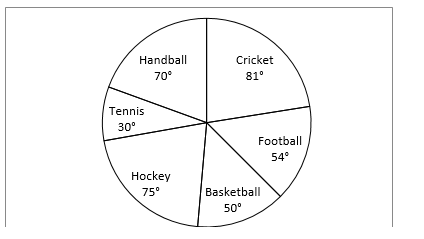Q6. Expenditure of India on handball is what percent more or less than the expenditure of India on Tennis and Basketball together.
(a) 15%
(b) 25%
(c) 12.5%
(d) 37.5%
(e) 10%

Q7. Total revenue from the Cricket and football is 100% more than the total expenditure on these two sports and it is equal to the total revenue occur on all other sports together. Find the ratio of profit occurred on cricket and football together to the profit occurred on all other sports?
(a) 2 : 1
(b) 1 : 3
(c) 2 : 3
(d) 3 : 1
(e) 4 : 3

Q8. If total expenditure on Hockey is 375 cr. Then find the difference of the expenditure on Handball and Tennis.
(a) 150 cr
(b) 250 cr
(c) 240 cr
(d) 100 cr
(e) 200 cr

Q9. Expenditure on Basketball is how much more than that that of Tennis if total expenditure is 2700 cr.
(a) 140 cr
(b) 150 cr
(c) 100 cr
(d) 120 cr
(e) 180 cr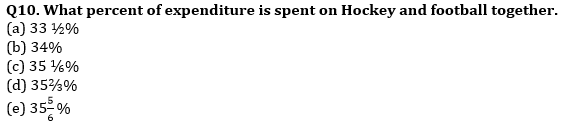Directions (11-15): study the following pie-chart carefully & answer the following questions.
Pie-chart given below shows the total number of passed candidates in different subjects in a class.
Total number of passed students = 12000

Q11. If the number of failed students in Hindi is 25% more than number of passed students in Hindi. Then number of failed students in Hindi is what percent of number of passed students in English?
(a) 80%
(b) 75%
(c) 55%
(d) 60%
(e) 70%

Q12. If total number of student who had taken exam of physics are 4000. Then find ratio of number of failed students in physics to number of passed students in chemistry?
(a) 11 : 6
(b) 13 : 6
(c) 11 : 5
(d) 3 : 2
(e) 2 : 1

Q13. If the total number of passed students are 25% of the total number of student in the class. Then total number of failed student is how much more/less than total number of passed students in computer, Math & Hindi together?
(a) 28,800
(b) 27,400
(c) 26,200
(d) 29,400
(e) 29,600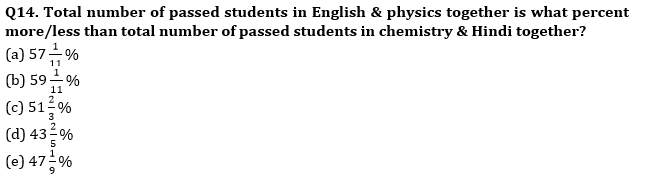Q15. If the ratio of total number of passed to total number of students in class is 2 : 5. And the percentage distribution of failed students is same as that of the passed students in the class. Then find average number of failed student in chemistry & English?
(a) 2200
(b) 3200
(c) 1500
(d) 1700
(e) 2700

Practice More Questions of Quantitative Aptitude for Competitive Exams:

###### SBI Clerk Study Plan 2021

Solutions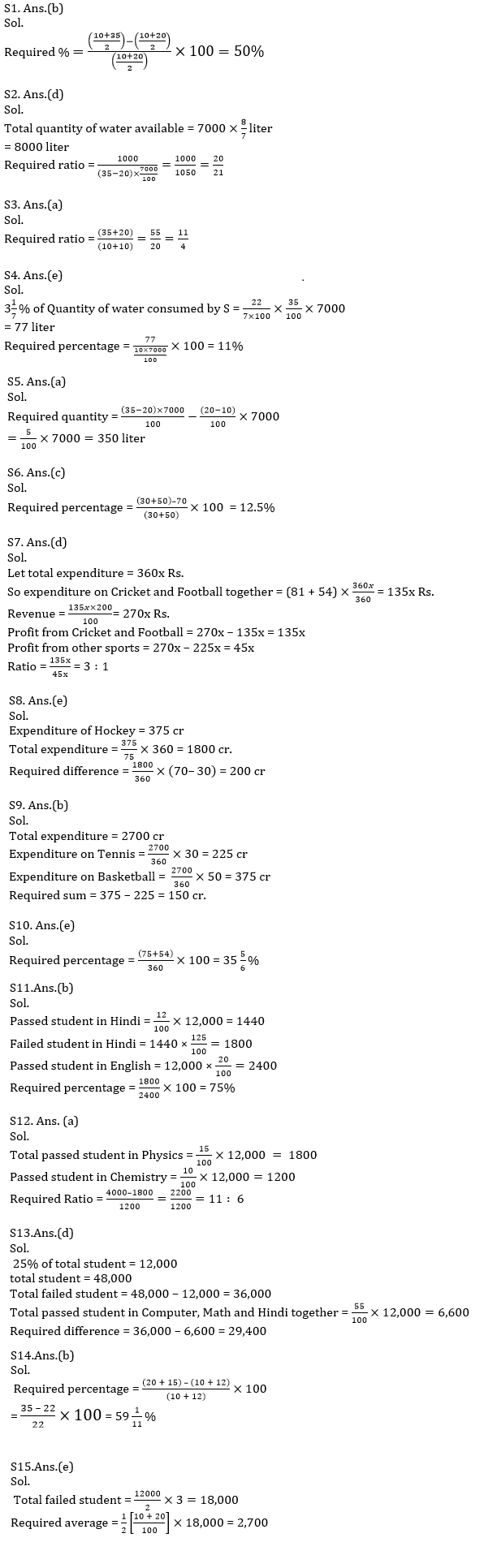#### Congratulations!